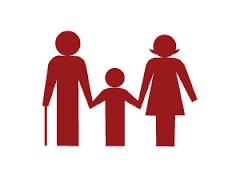# Birthday 3043

Linda and her uncle Slavo have a birthday the same day. A few years ago, Linda was 13, and her uncle was 45 years old. How many years ago was it today that Uncle Slavo was three times older than Linda?

x =  3

### Step-by-step explanation:

(45+x) = 3(13+x)

(45+x) = 3·(13+x)

2x = 6

x = 6/2 = 3

x = 3

Our simple equation calculator calculates it.Did you find an error or inaccuracy? Feel free to write us. Thank you!

Tips for related online calculators
Do you have a linear equation or system of equations and looking for its solution? Or do you have a quadratic equation?
Do you want to convert time units like minutes to seconds?